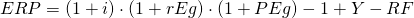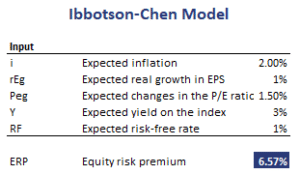## Ibbotson-Chen model

The Ibbotson-Chen model is a macroeconomic model for the Equity Risk Premium (ERP). Macroeconomic models are based on the relationship between macroeconomic variables and financial variables.

It is important to note that macroeconomic equity risk premium models are only appropriate for developed countries. That’s because in developed countries public equities represent a relatively large share of the economy. Thus, it is reasonable to assume that there may be a relationship between stock market performance and macroeconomic variables. The same may generally not hold true for developing countries. The main advantage of supply-side models is that they are based on proven macroeconomic models and current information.

The Ibbotson-Chen model, proposed in 2003, provides a supply-side estimate of the equity risk premium. On this page, we discuss the Ibbotson-Chen model definition as well as the definitions of the underlying variables. We also provide an example in Excel.

## Ibbotson-Chen earnings model formula

The Ibbotson-Chen model formula looks as followswhere i is the expected inflation, rEg is the expected real growth in EPS, PEg is the expected changes in the P/E ratio, Y is the expected yield on the index, and RF is the expected risk-free rate.

The individual components are determined as follows:

• Expected inflation is typically based on a market-based estimate of expected inflation, such as the spread between the yield on a T-bond and Treasury Inflation Protected Securities (TIPS).
• Expected real growth in EPS can be approximated as the real GDP growth rate
• Expected growth in the PE ratio is based on the analyst’s judgement of whether the market is overvalued or not
• Expected yield on the index can be estimated using the dividends on the index.

Once we have estimates for all the components, we can apply the model to calculate the equity risk premium.

## Ibbotson-Chen model example

Let’s apply the model to some actual data. The following table applies the model using estimates on the different components.## Summary

We discussed the supply side rate of return model or Ibbotson-Chen model which can be used to estimate the equity risk premium. Alternatives to this model include survey estimates and the Constant Growth model (or Gordon Growth model).

### Ibbotson-Chen

Want to have an implementation in Excel? Download the Excel file: Ibbotson-Chen